### Why should I choose AnalystNotes?

Simply put: AnalystNotes offers the best value and the best product available to help you pass your exams.

##### Subject 1. Accounting for Bond Issuance, Bond Amortization, Interest Expense, and Interest Payments
Debt is classified as short-term (ST) and long-term (LT).

• Current liabilities result from both operating and financing activities.

• Those caused by operating activities include accounts payable and advances from customers. Operating and trade debt is reported at the expected (undiscounted) cash flow and is an important exception to the rule that liabilities are recorded at present value. Note that advances from customers are the consequence of operating decisions, the result of normal activity. They should be distinguished from other payables when analyzing a firm's liquidity. Advances are a prediction of future revenues rather than cash outflows.

• Those resulting from financing activities include short-term (ST) debt and the current portion of long-term (LT) debt. They are recorded at present value. Note that the current portion of LT debt is the consequence of financing activity and indicates a need for cash or refinancing. A shift from operating to financing indicates the beginning of liquidity problems, and inability to repay ST credit is a sign of financial distress.

• Long-term debt results from financing activities. It may be obtained from many sources that may differ in interest and principal payments. Some claims are below or subordinated to others while other claims may be senior or have priority. Whatever the different payment terms are, there are two basic principles:

• Debt equals present value of the future interest and principal payments. For book values the discount rate is the rate when debt was incurred. For market values the discount rate is the current rate.

• Interest expense is the amount paid to the creditor in excess of the amount received. Though the total to be paid is known, allocation to specific time periods may be uncertain. The coupon rate is just the stated cash interest rate.

Below we will focus on debt resulting from financing activities.

• A bond premium represents the amount over the face value of the bond that the issuer never has to return to the bondholders. In effect it reduces the higher-than-market interest rate that the issuer is paying on the bond. It must be allocated over the life of the bond as a reduction of interest expense each period.
• A bond discount represents the amount in excess of the issue price that must be paid by the issuer at the time of maturity. In effect it increases the lower-than-market interest rate the issuer is paying on the bond. It must be allocated over the life of the bond as an increase of interest expense each period.

At the time of issuance, the firm receives proceeds from issuing the bond. A bond payable is valued at the present value of its future cash flows (periodic coupon payments and principal repayment at maturity). These cash flows are discounted at the market rate of interest at issuance. Therefore, the value of the bond depends on the market rate of interest. For example, if the market rate of interest is higher than the coupon rate, the bond value will be less than its face value, and the bond is issued at a discount.

• Balance sheet. Initial liability is the amount paid to the issuer by the lender. The amount may not equal to the face value of the bond.

• Issued at par on Interest Date:
If \$800,000 of bonds were issued on January 1, 2015 at 100, the issuance would be recorded as follows: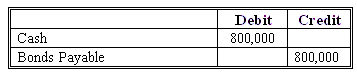• Issued at Discount on Interest Date:
If \$800,000 of bonds were issued on January 1, 2015 at 97, the issuance would be recorded as follows:• Issued at Premium on Interest Date:
If \$800,000 of bonds were issued on January 1, 2015 at 103, the issuance would be recorded as follows: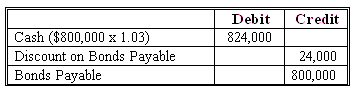• Cash flow statement. Cash flow from financing (CFF) increases by the amount received.

At the end of each semi-annual payment period, the firm makes a coupon payment:

• Income statement. Interest expense is reported here. The effective interest rate is the market rate at the time of issuance, and the interest expense is market rate multiplied by the bond liability at the beginning of this six-month period.

• Cash flow statement. Cash flow from operations (CFO) decreases by the coupon payment. The coupon rate and face value are used to calculate actual cash flows only.

• Balance sheet. The bond liability is adjusted if necessary. Liability over time is a function of (1) initial liability and the relationship of (2) interest expense to (3) the actual cash payments. That is, the difference between interest expense and coupon payment represents the change in bond liability during this period: change in bond liability = interest expense - coupon payment. The ending bond liability = beginning bond liability + change in bond liability.

The bond premium or discount is amortized over the life of the bond by what is known as the interest method. This results in a constant rate of interest (not a constant interest expense) over the life of the bond. Bond interest expense is increased by amortization of a discount and decreased by amortization of a premium.

• If the bond is issued at a premium, interest expense is always lower than coupon payment, and decreases over time. In this case the interest expense is only one component of the coupon payment. The rest of the coupon payment is used to amortize the bond's premium.
• If the bond is issued at a discount, interest expense is always higher than coupon payment, and increases over time. In this case the interest expense has two components: the coupon payment and amortization amount of the bond's discount.
• If the bond is issued at par, interest expense equals coupon payment.

At any point in time the liability on the balance sheet will equal the present value of the remaining cash flow payments to the creditor discounted at the effective market interest rate.

At the maturity date, the firm repays the face value of the bond. The treatment and effects of the last coupon payment are the same as those shown above.

• Balance sheet: the bond liability is reduced by the face value.
• Cash flow statement: similar to the treatment of initial cash received, the final face value payment is treated as CFF.

Total interest expense is equal to amounts paid by the issuer to the creditor in excess of the amount received.

Summary of the Effective Interest Method

• Bond interest expense is computed first by multiplying the carrying value of the bonds at the beginning of the period by the effective interest rate:

Interest Expense = Beginning Carrying Value x Market Rate of Interest

• The bond discount or premium amortization is then determined by comparing the bond interest expense with the interest to be paid. Note that Interest Payment = Face Value x Coupon Rate x 1/2 (assume semi-annual coupon payment).

• The carrying value of the bond at the end of the period = Beginning Carrying Value - Amortization of Bond Premium (or + Amortization of Bond Discount).

This method produces a periodic interest expense equal to a constant percentage of the carrying value of the bonds. Since the percentage is the effective rate of interest incurred by the borrower at the time of issuance, the effective interest method results in a better matching of expense with revenues than the straight-line method.

Example

Evermaster Corporation issued \$100,000 of 8% term bonds on January 1, 2015, due on January 1, 2020, with interest payable each July 1 and January 1. Since investors required an effective interest rate of 10%, they paid \$92,278 for the \$100,000 of bonds, creating a \$7722 discount.

1. To record the issuance: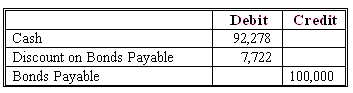2. To record the first interest payment of \$4,000 on July 1, 2015:Note: 92,278 x 0.10 x 0.5 = \$4,614. Now the discount balance is 7,722 - 614 = \$7,108.

3. To record the second interest payment of \$4,000 on January 1, 2016: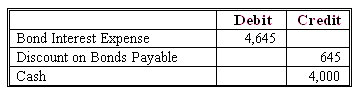Note: (100,000 - 7,108) x 0.10 x 0.5 = \$4,645. Now the discount balance is 7,108 - 645 = \$6,463.

And so on.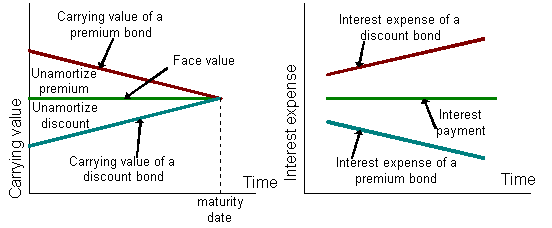• The carrying value of a premium (discount) bond decreases (increases) over time.
• At the maturity date, the carrying value of both a premium bond and a discount bond equals the face value.
• The interest expense of a discount (premium) bond increases (decreases) over time due to the increasing (decreasing) carrying value.

Zero-Coupon Bond

Zero-coupon bond has no periodic interest payments and is issued at a large discount from par. The proceeds at issuance equal the present value of the face value, discounted at the market value of interest at issuance. Repayment at maturity includes all the (implied) interest expense (equal to face value minus the proceeds) from the time of issuance: Total Implied Interest = Par Value - Proceeds Received.

In essence, zero-coupon bonds are a special type of discount bonds. Therefore, their effects on financial statements are similar to those of discount bonds.

• The interest expense on a zero-coupon bond never reduces operating cash flow. Reported CFO is systematically "overstated" when a zero-coupon (or deep-discount) bond is issued, while CFF is understated by the amortization amount of the discount and should be adjusted accordingly.
• Unlike discount bonds (whose reported CFO is reduced by the coupon payments), they make no coupon payments so they have no effect on reported CFO.
• Solvency ratios, such as cash-basis interest coverage, are improved relative to the issuance of par bonds. The cash eventually required to repay the obligations may become a significant burden.

For example, assume a market interest rate of 5%, a \$100,000 face value zero-coupon bond payable in three years will be issued at \$86,229.68 (semi-annual compounding). This is computed by pressing the following calculator keys: 100000 FV; 2.5I/Y; 6N; CPT PV.

The journal entry (of the issuer) to record this issue will be:
Bank (balance sheet): \$86,229.68 (debit)
Bond liability (balance sheet): \$86,229.68 (credit).

When the bond is paid at maturity, the repayment of \$100,000 includes \$13,770.32 of interest. The major difference between a zero-coupon and a par value bond is that the interest of \$13,770.32 is never reported as a cash flow from operations for a zero-coupon bond.

This is because the full \$100,000 is reported as a cash flow from financing.

User Comment
teddajr if MR > CR => PRM else if CR > MR => DISC
leo6fin I think its the other way around

if MR > CR => DISC else MR < CR => PRM
fanfanli Can someone explain why an inflow of cash, is accounted for as a Debit here.

Would this not be a credit to the asset side (cash) and a credit to the liability side (Bond Payable).
TMP1982 fanfanil everything must equal in the accounting equation. an addition to cash on the asset side is a debit. Debit has no negative or positive implications in accounting- just means left or right (think T diagrams). I hope this helps.
Nitishm In the example above, how did they get \$92278 as the amount that investors will pay for the bonds. What is the calculation to get to that figure?
gill15 I'm soo happy to not be taxing right now.
Kevdharr Same here gill15. And Nitishm, the \$92,278 is derived by calculating the present value of each coupon payment discounted at the market rate of interest (10% in this example) and adding it to the present value of the principal repayment of \$100,000 (also discounted at 10%). When you add those numbers together, you should get \$92,278 or something close to it.
degosan9 I keep doing the above calculation but I keep getting a different number. I keep getting \$92418. That's five \$8k payments discounted back five years at 10% and one \$100k payments discounted back five years at 10%. What am I missing?
degosan9 Nevermind. I'm a dummy. Was doing 10% annual x 5 instead of 5% semiannual x 10
aguest why CFO will decrease after coupon payment? Isn't it part of cash flow from financing (CFF)?
aguest interest payments are part of CFO
sanyukta Trick for remembering Debits vs Credits: think of DEAD.

Debits increase Expenses, Assets, and Dividends. All else has credit balance
breh use this to remember Debits vs. Credits. DEAD. Debits increase Expenses Assets and Dividends. Everything else has a credit balance. So cash inflow would should be considered a debit, as it increases Assets.